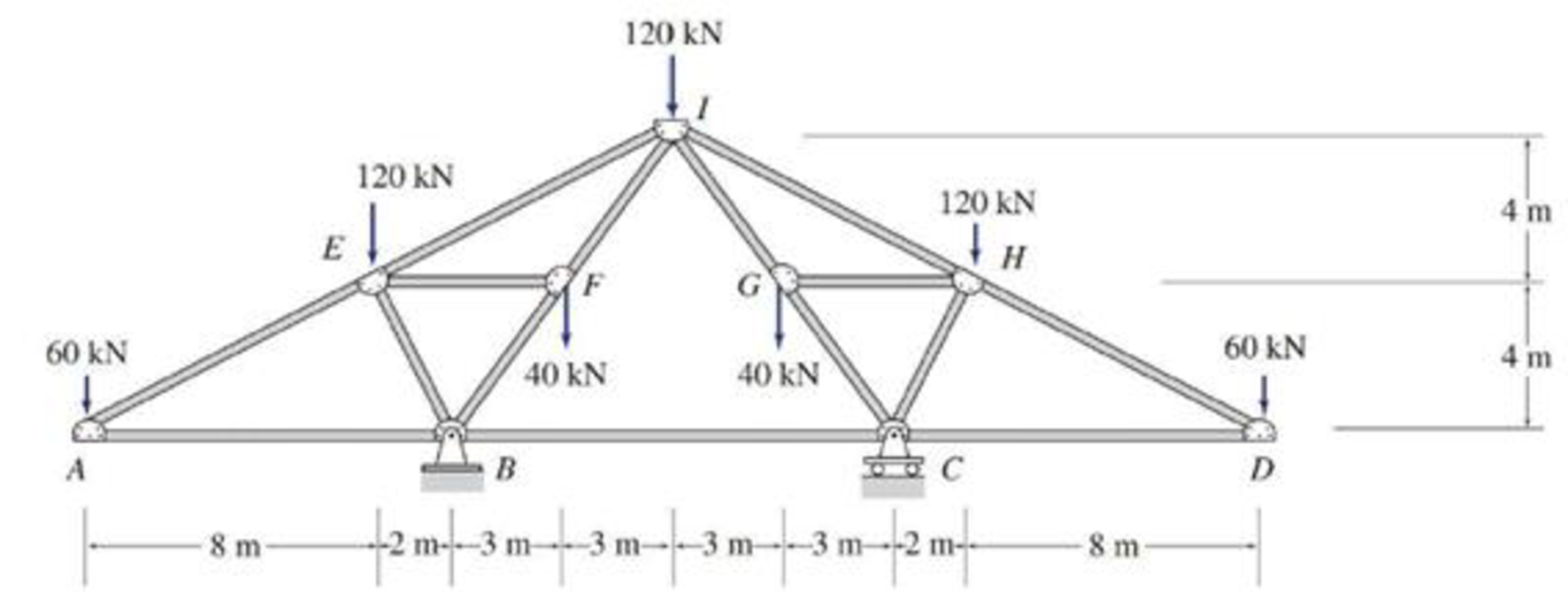# 4.46 through 4.50 Determine the force in each member of the truss shown. FIG. P4.47

#### Solutions

Chapter
Section
Chapter 4, Problem 47P
Textbook Problem
765 views

## 4.46 through 4.50 Determine the force in each member of the truss shown.FIG. P4.47

To determine

Find the forces in the members of the truss.

### Explanation of Solution

Given information:

Apply the sign conventions for calculating reactions, forces and moments using the three equations of equilibrium as shown below.

• For summation of forces along x-direction is equal to zero (Fx=0), consider the forces acting towards right side as positive (+) and the forces acting towards left side as negative ().
• For summation of forces along y-direction is equal to zero (Fy=0), consider the upward force as positive (+) and the downward force as negative ().
• For summation of moment about a point is equal to zero (Matapoint=0), consider the clockwise moment as negative and the counter clockwise moment as positive.

Method of joints and section.

The negative value of force in any member indicates compression (C) and the positive value of force in any member indicates Tension (T).

Calculation:

Show the free body diagram of the truss as shown in Figure 1.

Refer Figure 1.

Consider the horizontal and vertical reactions at B are Bx and By.

Consider the vertical reaction at C is Cy.

Take the sum of the forces in the horizontal direction as zero.

Fx=0Bx=0

Take the sum of the forces in the vertical direction as zero.

By the symmetry of truss,

Fy=0By=Cy=(60+120+120+120+602)By=Cy=(60+120+40+120+40+120+602)By=Cy=280kN

Calculate the value of the angle θ as follows:

tanθ=(816)θ=tan1(816)θ=26.565°

Calculate the value of the angle α as follows:

tanα=(42)α=tan1(2)α=63.434°

Calculate the value of the angle β as follows:

tanβ=(86)β=tan1(86)β=53.130°

Consider a section a-a passing through the members BC, CG, and JK.

Show the portion of the truss to the left of the section a-a as shown in Figure 2.

Refer Figure 2.

Take the sum of the moment about I as zero.

MF=060×16120×840×3+280×6FBC×8=0FBC=3608FBC=45kN(C)

JOINT A.

Show the joint as shown in Figure 3.

Refer Figure 3.

Find the force in the member AE and AB as follows:

For Equilibrium of forces,

Fy=0FAEsin26.565°=60FAE=60sin26.565°FAE=134.16kN(T)

Fx=0FAEcos26.565°+FAB=0FAB=FAEcos26.565°FAB=120kNFAB=120kN(C)

JOINT B.

Show the joint as shown in Figure 4.

Refer Figure 4.

Find the forces in the members BE and BF as follows:

For Equilibrium of forces,

Fx=0FBEcos63.434°+FBFcos53.130°FBA+FBC=00

### Still sussing out bartleby?

Check out a sample textbook solution.

See a sample solution

#### The Solution to Your Study Problems

Bartleby provides explanations to thousands of textbook problems written by our experts, many with advanced degrees!

Get Started

Find more solutions based on key concepts
VocabularyState the meaning of the following terms: Dimensional Homogeneity _____ Unit Conversion _____

Engineering Fundamentals: An Introduction to Engineering (MindTap Course List)

What are two different ways that apprenticeships are structured?

Precision Machining Technology (MindTap Course List)

What is the difference between a cybercriminal and a cyberterrorist?

Principles of Information Systems (MindTap Course List)

Convert 5 megawatts of power into BTU/hr, ft-lbs/s, and kJ/hr.

Fundamentals of Chemical Engineering Thermodynamics (MindTap Course List)

Write the SQL code that will save the changes made to the EMP_1 table.

Database Systems: Design, Implementation, & Management

Identify features of Windows. Define the term, desktop, with respect to Windows and Mac OS.

Enhanced Discovering Computers 2017 (Shelly Cashman Series) (MindTap Course List)

What types of injuries can occur to the ears during welding?

Welding: Principles and Applications (MindTap Course List)

If your motherboard supports ECC DDR3 memory, can you substitute non-ECC DDR3 memory?

A+ Guide to Hardware (Standalone Book) (MindTap Course List)